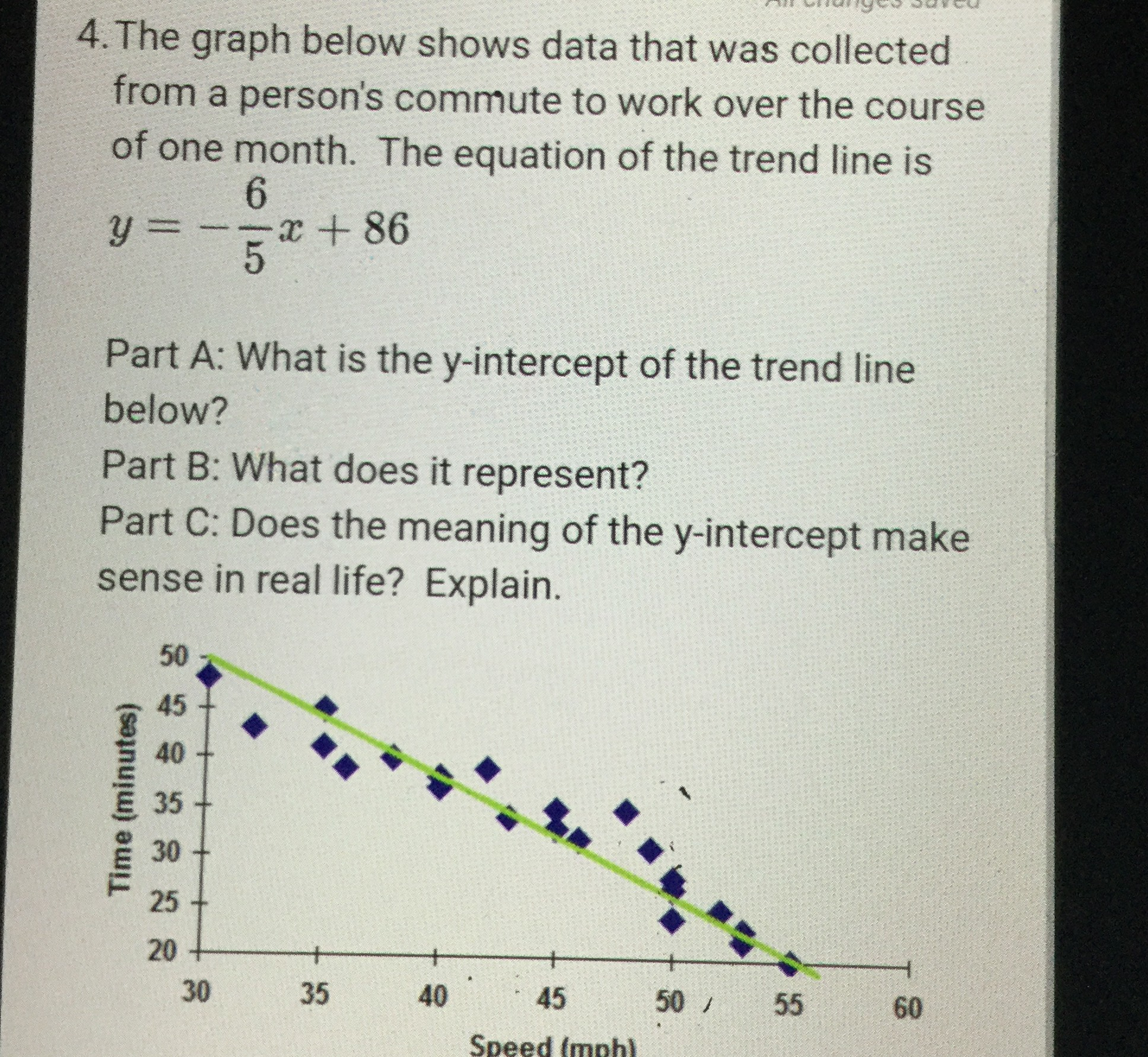### ¿Todavía tienes preguntas de matemáticas?

Pregunte a nuestros tutores expertos
Algebra
Pregunta4. The graph below shows data that was collected from a person's commute to work over the course of one month. The equation of the trend line is

$$y = - \frac { 6 } { 5 } x + 86$$

Part A: What is the $$y$$ -intercept of the trend line below? Part B: What does it represent? Part C: Does the meaning of the $$y$$ -intercept make sense in real life? Explain.